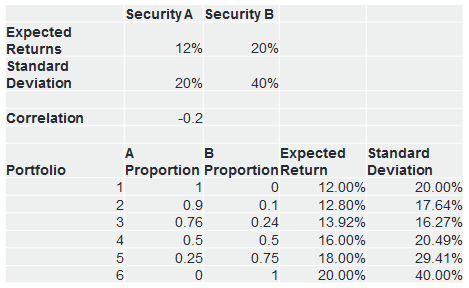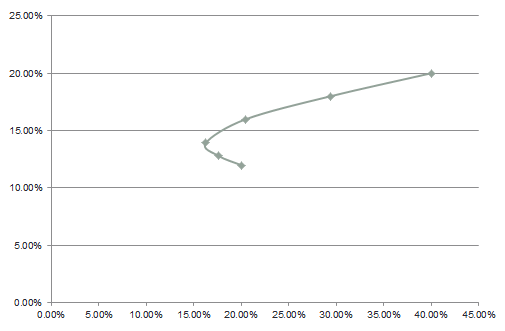# Efficient Frontier for a Portfolio of Two Assets

We learned that the calculation of risk for a portfolio of two assets is not straight forward as we also have to account for the covariance between the assets in the portfolio.

Depending on the correlation between the assets, the risk-return profile of the portfolio changes. Note that we can combine the two assets in varying proportions in the portfolio two arrive at an infinite number of portfolios. Say the two assets are A and B. You can start with a portfolio that has 100% money invested in Stock A, then create many portfolios with different proportions of A and B, and end with a portfolio that has 100% money invested in stock B.

When you plot the risk-return profile of all these portfolios, what you see is the feasible set of portfolios.

Let’s take an example to understand this. Let’s say we have two securities A and B with the following expected returns, standard deviation, and correlation. Below you will also see 6 different portfolios with varying proportions of A and B.Portfolio of Two Assets

When we plot the risk-return profile of these portfolios, it looks as follows:Efficient Frontier

Notice that there is a minimum variance portfolio with lowest risk on the efficient frontier.

We can make the following observations from this:

• There are benefits of diversification, as the risk reduces when we combine assets in the portfolio.
• There is a Minimum Variance Portfolio (Portfolio 3) that has the minimum risk.
• The feasible set or opportunity set is represented by the entire curved line.
• The curve bends backwards.
• Investors invest above the Minimum Variance Portfolio (MVP), as any portfolio below it does not have an optimal risk-return profile.

Get our Data Science for Finance Bundle for just $29$51.